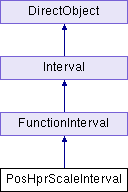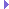PosHprScaleInterval Class Reference
Inheritance diagram for PosHprScaleInterval:## Public Member Functions

def __init__ (self, nodePath, pos, hpr, scale, duration=0.0, name=None, other=None)Public Member Functions inherited from FunctionInterval
def __init__ (self, function, **kw)

def privInstant (self)

def replaceMethod (self, oldFunction, newFunction)Public Member Functions inherited from Interval
def __init__ (self, name, duration, openEnded=1)

def __repr__ (self, indent=0)

def clearToInitial (self)

def finish (self)

def getDoneEvent (self)

def getDuration (self)

def getLoop (self)

def getName (self)

def getOpenEnded (self)

def getPlayRate (self)

def getState (self)

def getT (self)

def intervalDone (self)

def isPaused (self)

def isPlaying (self)

def isStopped (self)

def loop (self, startT=0.0, endT=-1.0, playRate=1.0)

def pause (self)

def popupControls (self, tl=None)

def privDoEvent (self, t, event)

def privFinalize (self)

def privInitialize (self, t)

def privInterrupt (self)

def privPostEvent (self)

def privReverseFinalize (self)

def privReverseInitialize (self, t)

def privReverseInstant (self)

def privStep (self, t)

def resume (self, startT=None)

def resumeUntil (self, endT)

def setDoneEvent (self, event)

def setLoop (self, loop=1)

def setPlayRate (self, playRate)

def setT (self, t)

def setupPlay (self, startT, endT, playRate, doLoop)

def setupResume (self)

def start (self, startT=0.0, endT=-1.0, playRate=1.0)

def stepPlay (self)Public Member Functions inherited from DirectObject
def __init__ (self)

def accept (self, event, method, extraArgs=[])

def acceptOnce (self, event, method, extraArgs=[])

def addTask (self, *args, **kwargs)

def detectLeaks (self)

def doMethodLater (self, *args, **kwargs)

def getAllAccepting (self)

def ignore (self, event)

def ignoreAll (self)

def isAccepting (self, event)

def isIgnoring (self, event)

def removeAllTasks (self)

def removeTask (self, taskOrName)

## Static Public Attributes

int posHprScaleIntervalNum = 1Static Public Attributes inherited from FunctionInterval
int functionIntervalNum = 1

FunctionIntervals = weakref.WeakKeyDictionary()

notify = directNotify.newCategory('FunctionInterval')Static Public Attributes inherited from Interval
notify = directNotify.newCategory("Interval")

int playbackCounter = 0Static Public Attributes inherited from DirectObject
def accept_once = acceptOnce

def add_task = addTask

def detect_leaks = detectLeaks

def do_method_later = doMethodLater

def get_all_accepting = getAllAccepting

def ignore_all = ignoreAll

def is_accepting = isAccepting

def is_ignoring = isIgnoring

def remove_all_tasks = removeAllTasks

def remove_task = removeTask

## Additional Inherited MembersStatic Public Member Functions inherited from FunctionInterval
def makeUniqueName (func, suffix='')Public Attributes inherited from FunctionInterval
extraArgs

function

kwPublic Attributes inherited from Interval
currT

doneEvent

duration

es

name

openEnded

pname

pstats

setTHooks

stateProperties inherited from Interval
done_event = property(getDoneEvent, setDoneEvent)

open_ended = property(getOpenEnded)

play_rate = property(getPlayRate, setPlayRate)

stopped = property(isStopped)

t = property(getT, setT)

## ◆ __init__()

 def __init__ ( self, nodePath, pos, hpr, scale, duration = `0.0`, name = `None`, other = `None` )
```__init__(nodePath, pos, hpr, scale, duration, other, name)
```

## ◆ posHprScaleIntervalNum

 int posHprScaleIntervalNum = 1
static